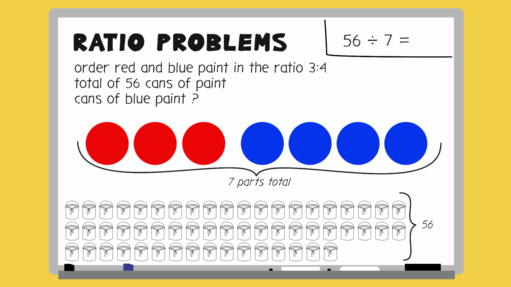Everyday maths 2 (Wales)

Start this free course now. Just create an account and sign in. Enrol and complete the course for a free statement of participation or digital badge if available.

# 10.2 Solving ratio problems where the total is given

The best way for you to understand how to solve these problems is to look through the worked example in the video below.Interactive feature not available in single page view (see it in standard view).

## Activity 25: Ratio problems where the total is known

Try solving these ratio problems:

1. To make mortar you need to mix soft sand and cement in the ratio 4:1. You need to make a total of 1500 g of mortar.

How much soft sand will you need?

1. Add the parts of the ratio:

• 4 + 1 = 5

Divide the total amount required by the sum of the parts of the ratio:

• 1500 g ÷ 5 = 300 g

Since soft sand is 4 parts, we do 300 g × 4 = 1200 g of soft sand.

Check by working out how much cement you need. Cement is 1 part so you would need 300 g:

• 1200 g + 300 g = 1500 g which is the correct total.
1. To make the mocktail ‘Sea Breeze’ you need to mix cranberry juice and grapefruit juice in the ratio 4:2.

You want to make a total of 2700 ml of mocktail. How much grapefruit juice should you use?

1. Add the parts of the ratio:

• 4 + 2 = 6

Divide the total amount required by the sum of the parts of the ratio:

• 2700 ml ÷ 6 = 450 ml

Since grapefruit juice is 2 parts, we do 450 ml × 2 = 900 ml of grapefruit juice.

Check by working out how much cranberry juice you would use:

• 4 × 450 = 1800

1800 ml + 900 ml = 2700 ml

You may have simplified the ratio to 2:1 before doing the calculation, but you will see that your answers are the same as ours.

1. The instructions to mix Misty Morning paint are mix 150 ml of azure with 100 ml of light grey and 250 ml of white paint.

How much light grey paint would you need to make 5 litres of Misty Morning?

1. Start by expressing and then simplifying the ratio:

• 150:100:250 which simplifies to 3:2:5 = 10 parts
• 5 litres = 5000 ml (converting to ml makes your calculation easier.)

Divide the total amount required by the sum of the parts of the ratio:

• 5000 ÷ 10 = 500 so 1 part = 500 ml
• Light grey is 2 parts:

2 × 500 = 1000 ml or 1 litre

Check:

• azure is 3 parts: 3 × 500 = 1500 ml or 1.5 litres
• white is 5 parts: 5 × 500 = 2500 ml or 2.5 litres
• 1000 + 1500 + 2500 = 5000 ml or 5 litres
1. You want to make 14 litres of squash for a children’s party. The concentrate label says mix with water in the ratio of 2:5.

How much concentrate will you use?

1. Add the parts of the ratio:

• 2 + 5 = 7

Divide the total amount required by the sum of the parts of the ratio:

• 14 litres ÷ 7 = 2 litres so 1 part = 2 litres

(Note: this calculation was straightforward so there was no need to convert to ml.)

Since the concentrate is 2 parts you will need 2 litres × 2 = 4 litres of concentrate.

Check:

• Water is 5 parts:
•      5 × 2 litres = 10 litres
• 4 + 10 = 14 litres.
1. A man leaves £8400 in his will to be split between 3 charities:

• Dogs Trust, RNLI and MacMillan Research in the ratio 3:2:1.

How much will each charity receive?

1. Add the parts of the ratio:

• 3 + 2 + 1 = 6

Divide the total amount required by the sum of the parts of the ratio:

• £8400 ÷ 6 = 1400
•   –  The Dogs Trust receives 3 parts: 3 × £1400 = £4200
•   –  The RNLI receives 2 parts: 2 × £1400 = £2800
•   –  MacMillan Research receives 1 part so: £1400
• Check:

4200 + 2800 + 1400 = £8400

Next you’ll look at ratio problems where the total of one part of the ratio is known.#### Find out more

*Eligibility rules apply for financial support.